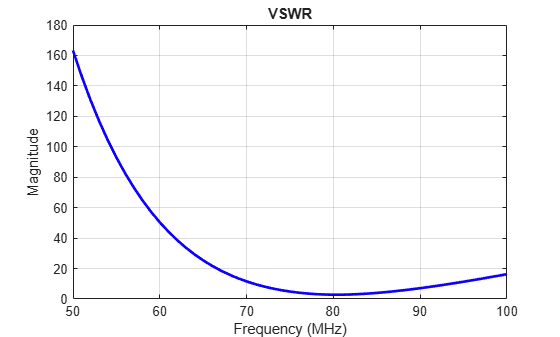vswr

Voltage standing wave ratio of antenna

Description

example

vswr(antenna,frequency,z0) calculates and plots the voltage standing wave ratio of an antenna, over specified frequency range, and given reference impedance, z0.

example

vswrant = vswr(antenna,frequency,z0) returns the VSWR of the antenna.

Examples

collapse all

Plot vswr (voltage standing wave ratio) of a circular loop antenna.

h = loopCircular;
vswr(h,50e6:1e6:100e6,50)Calculate vswr (voltage standing wave ratio) of a helix antenna.

h = helix;
hvswr = vswr(h,2e9:1e9:4e9,50)
hvswr = 1×3

3.5981    6.7141    3.3519

Input Arguments

collapse all

Antenna object, specified as a scalar.

Frequency range used to calculate VSWR, specified as a vector in Hz. The minimum value of frequency must be 1 kHz.

Example: 50e6:1e6:100e6

Data Types: double

Reference impedance, specified as a scalar in ohms.

Output Arguments

collapse all

Voltage standing wave ratio, returned as a vector in dB.